# Area FormulasPage 1

#### WATCH ALL SLIDES

Slide 1Area Formulas

Slide 2## Rectangle

Slide 3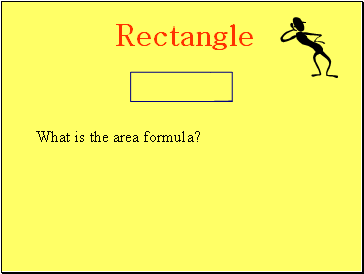Rectangle

What is the area formula?

Slide 4Rectangle

What is the area formula?

bh

Slide 5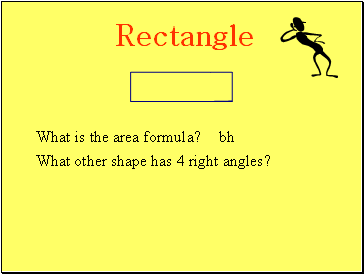Rectangle

What is the area formula?

bh

What other shape has 4 right angles?

Slide 6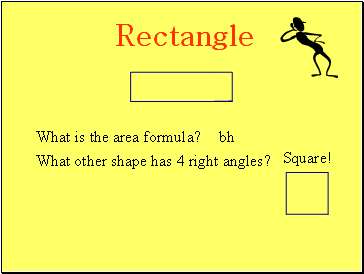Rectangle

What is the area formula?

bh

What other shape has 4 right angles?

Square!

Slide 7Rectangle

What is the area formula?

bh

What other shape has 4 right angles?

Square!

Can we use the same

area formula?

Slide 8Rectangle

What is the area formula?

bh

What other shape has 4 right angles?

Square!

Can we use the same

area formula?

Yes

Slide 9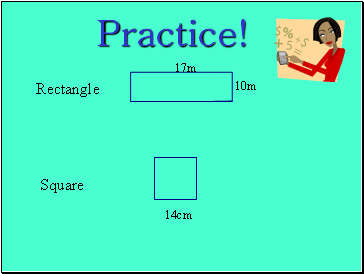Practice!

Rectangle

Square

10m

17m

14cm

Slide 10Rectangle

Square

10m

17m

14cm

196 cm2

170 m2

Slide 11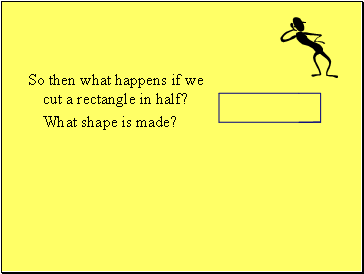So then what happens if we cut a rectangle in half?

Slide 12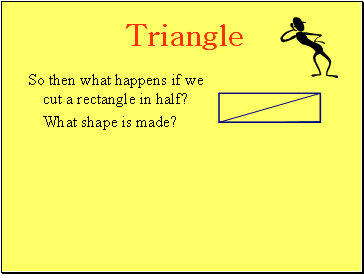## Triangle

So then what happens if we cut a rectangle in half?

Slide 13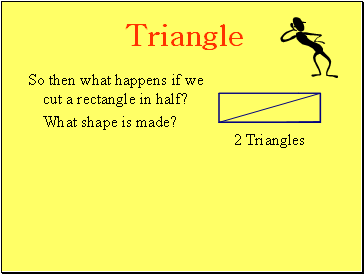Triangle

So then what happens if we cut a rectangle in half?

2 Triangles

Slide 14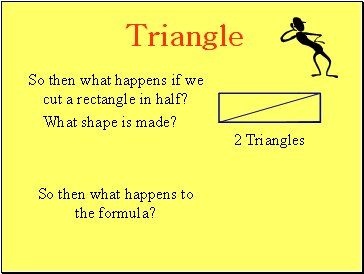Triangle

So then what happens if we cut a rectangle in half?

2 Triangles

So then what happens to the formula?

Slide 15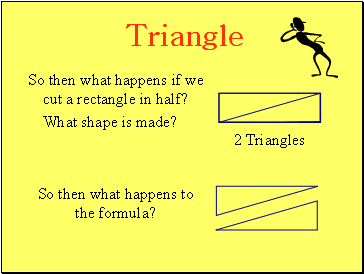Triangle

So then what happens if we cut a rectangle in half?

2 Triangles

So then what happens to the formula?

Slide 16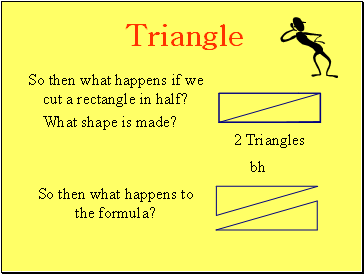Triangle

Go to page:
1  2  3  4  5  6  7